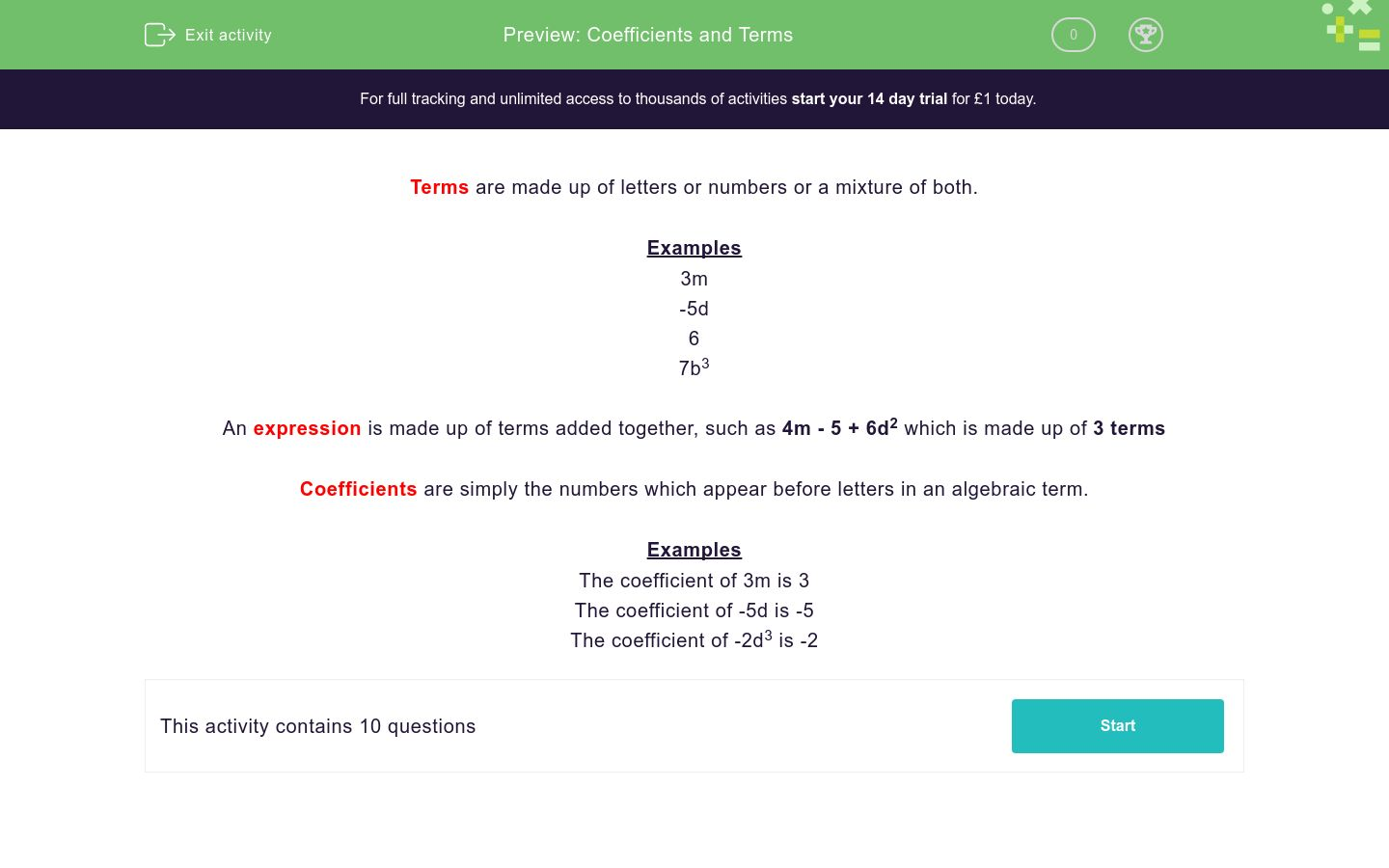# Coefficients and Terms

In this worksheet, students identify coefficients and terms in algebraic expressions.Key stage:  KS 3

Curriculum topic:   Algebra

Curriculum subtopic:   Understand Expressions, Equations, Inequalities, Terms and Factors

Difficulty level:### QUESTION 1 of 10

Terms are made up of letters or numbers or a mixture of both.

Examples

3m

-5d

6

7b3

An expression is made up of terms added together, such as 4m - 5 + 6d2 which is made up of 3 terms

Coefficients are simply the numbers which appear before letters in an algebraic term.

Examples

The coefficient of 3m is 3

The coefficient of -5d is -5

The coefficient of -2d3 is -2

How many terms are there in this expression?

5 - 5a

What is the coefficient of b in this expression?

5b - 5a + 3

5b

5

-5

3

How many terms are there in this expression?

5 - 5a + a2

How many terms are there in this expression?

-5a

What is the coefficient of b in this expression?

7b - 5b2 + 3

7b

-5

7

3

What is the coefficient of b2 in this expression?

7b - 5b2 + 3

-5b

-5

5

3

How many terms are there in this expression?

5 - 5b +2a + a2

How many terms are there in this expression?

-5a + 7a

What is the coefficient of b in this expression?

7c - 5b2 + 3b - 4

3b

-5

7

3

What is the coefficient of a in this expression?

7c - 5b2 + 3b - 4a

3b -

-4

-4a

4

• Question 1

How many terms are there in this expression?

5 - 5a

2
• Question 2

What is the coefficient of b in this expression?

5b - 5a + 3

5
• Question 3

How many terms are there in this expression?

5 - 5a + a2

3
• Question 4

How many terms are there in this expression?

-5a

1
• Question 5

What is the coefficient of b in this expression?

7b - 5b2 + 3

7
• Question 6

What is the coefficient of b2 in this expression?

7b - 5b2 + 3

-5
• Question 7

How many terms are there in this expression?

5 - 5b +2a + a2

4
• Question 8

How many terms are there in this expression?

-5a + 7a

2
• Question 9

What is the coefficient of b in this expression?

7c - 5b2 + 3b - 4

3
• Question 10

What is the coefficient of a in this expression?

7c - 5b2 + 3b - 4a

-4
---- OR ----

Sign up for a £1 trial so you can track and measure your child's progress on this activity.

### What is EdPlace?

We're your National Curriculum aligned online education content provider helping each child succeed in English, maths and science from year 1 to GCSE. With an EdPlace account you’ll be able to track and measure progress, helping each child achieve their best. We build confidence and attainment by personalising each child’s learning at a level that suits them.

Get started